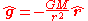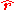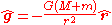xGravitational accelerationEncyclopedia
In physics
Physics
Physics is a natural science that involves the study of matter and its motion through spacetime, along with related concepts such as energy and force. More broadly, it is the general analysis of nature, conducted in order to understand how the universe behaves.Physics is one of the oldest academic...

, gravitational acceleration is the acceleration
Acceleration
In physics, acceleration is the rate of change of velocity with time. In one dimension, acceleration is the rate at which something speeds up or slows down. However, since velocity is a vector, acceleration describes the rate of change of both the magnitude and the direction of velocity. ...

on an object caused by gravity. Neglecting friction such as air resistance, all small bodies accelerate in a gravitational field
Gravitational field
The gravitational field is a model used in physics to explain the existence of gravity. In its original concept, gravity was a force between point masses...

at the same rate relative to the center of mass.
This equality is true regardless of the masses or compositions of the bodies. At different points on Earth, objects fall with an acceleration between 9.78 and 9.82 m/s2 depending on latitude
Latitude
In geography, the latitude of a location on the Earth is the angular distance of that location south or north of the Equator. The latitude is an angle, and is usually measured in degrees . The equator has a latitude of 0°, the North pole has a latitude of 90° north , and the South pole has a...

, with a conventional
Convention (norm)
A convention is a set of agreed, stipulated or generally accepted standards, norms, social norms or criteria, often taking the form of a custom....

standard value
Standard gravity
Standard gravity, or standard acceleration due to free fall, usually denoted by g0 or gn, is the nominal acceleration of an object in a vacuum near the surface of the Earth. It is defined as precisely , or about...

of exactly 9.80665 m/s2 (approx. 32.174 ft/s2). Objects with low densities do not accelerate as rapidly due to buoyancy and air resistance.

The barycentric
Center of mass
In physics, the center of mass or barycenter of a system is the average location of all of its mass. In the case of a rigid body, the position of the center of mass is fixed in relation to the body...

gravitational acceleration at a point in space is given by:where:
M is the mass of the attracting object,is the unit vector from center of mass of the attracting object to the center of mass of the object being accelerated.
r is the distance between the two objects.
G is the gravitational constant
Gravitational constant
The gravitational constant, denoted G, is an empirical physical constant involved in the calculation of the gravitational attraction between objects with mass. It appears in Newton's law of universal gravitation and in Einstein's theory of general relativity. It is also known as the universal...

of the universe.

The relative acceleration of two objects in the reference frame of either object or the center of mass is:Thus, for a given total mass, relative gravitational acceleration does not depend on each mass separately. As long as one mass is much smaller than the other, relative gravitational acceleration is almost independent of the smaller mass.

All small masses brought in from far away and dropped one at a time will experience the same acceleration, relative to an inertial frame or the frame of the large mass. Disregarding air resistance, all small masses dropped simultaneously from the same height will hit the ground at the same time; for example, during Apollo 15
Apollo 15
Apollo 15 was the ninth manned mission in the American Apollo space program, the fourth to land on the Moon and the eighth successful manned mission. It was the first of what were termed "J missions", long duration stays on the Moon with a greater focus on science than had been possible on previous...

an astronaut on the Moon simultaneously dropped a feather and a hammer and they reached the ground at the same time.

## In general relativity

In Einstein's theory of general relativity
General relativity
General relativity or the general theory of relativity is the geometric theory of gravitation published by Albert Einstein in 1916. It is the current description of gravitation in modern physics...

, gravitation is an attribute of curved spacetime
Spacetime
In physics, spacetime is any mathematical model that combines space and time into a single continuum. Spacetime is usually interpreted with space as being three-dimensional and time playing the role of a fourth dimension that is of a different sort from the spatial dimensions...

instead of being due to a force propagated between bodies. In Einstein's theory, masses distort spacetime in their vicinity, and other particles move in trajectories determined by the geometry of spacetime. The gravitational force is a fictitious force. There is no gravitational acceleration, in that the proper acceleration
Proper acceleration
In relativity theory, proper acceleration is the physical acceleration experienced by an object. It is acceleration relative to a free-fall, or inertial, observer who is momentarily at rest relative to the object being measured...

and hence four-acceleration
Four-acceleration
In special relativity, four-acceleration is a four-vector and is defined as the change in four-velocity over the particle's proper time:whereandand \gamma_u is the Lorentz factor for the speed u...

of objects in free fall
Free fall
Free fall is any motion of a body where gravity is the only force acting upon it, at least initially. These conditions produce an inertial trajectory so long as gravity remains the only force. Since this definition does not specify velocity, it also applies to objects initially moving upward...

are zero. Rather than undergoing an acceleration, objects in free fall travel along straight lines (geodesics
Geodesic (general relativity)
In general relativity, a geodesic generalizes the notion of a "straight line" to curved spacetime. Importantly, the world line of a particle free from all external, non-gravitational, force is a particular type of geodesic...

) on the curved spacetime.

• Newton's law of universal gravitation
Newton's law of universal gravitation
Newton's law of universal gravitation states that every point mass in the universe attracts every other point mass with a force that is directly proportional to the product of their masses and inversely proportional to the square of the distance between them...

• Air track
Air Track
An air track is a scientific device used to study motion in low friction environment. Its name comes from its structure: air is pumped through a hollow track with fine holes all along the track that allows specially fitted air track cars to glide relatively friction-free. Air tracks are usually...

• Standard gravity
Standard gravity
Standard gravity, or standard acceleration due to free fall, usually denoted by g0 or gn, is the nominal acceleration of an object in a vacuum near the surface of the Earth. It is defined as precisely , or about...

• Gravity of Earth
• Gravimetry
Gravimetry
Gravimetry is the measurement of the strength of a gravitational field. Gravimetry may be used when either the magnitude of gravitational field or the properties of matter responsible for its creation are of interest...